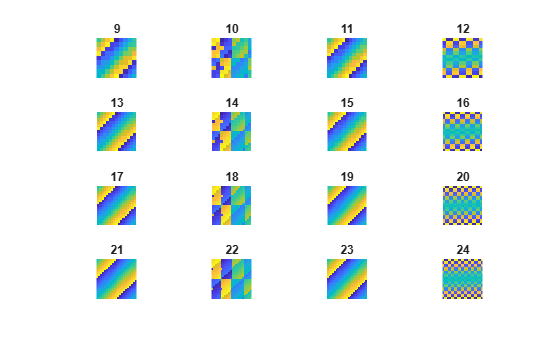# magic

## 语法

``M = magic(n)``

## 说明

``M = magic(n)` 返回由 `1` 到 `n`2 的整数构成并且总行数和总列数相等的 `n`×`n` 矩阵。`n` 的阶数必须是大于或等于 `3` 的标量才能创建有效的幻方矩阵。`

## 示例

`M = magic(3)`
```M = 3×3 8 1 6 3 5 7 4 9 2 ```

`sum(M)`
```ans = 1×3 15 15 15 ```
`sum(M,2)`
```ans = 3×1 15 15 15 ```

```for n = 1:16 subplot(4,4,n) ord = n+8; m = magic(ord); imagesc(m) title(num2str(ord)) axis equal axis off end```